# Test: Name Reaction- 2

## 30 Questions MCQ Test Organic Chemistry | Test: Name Reaction- 2

Description
This mock test of Test: Name Reaction- 2 for Chemistry helps you for every Chemistry entrance exam. This contains 30 Multiple Choice Questions for Chemistry Test: Name Reaction- 2 (mcq) to study with solutions a complete question bank. The solved questions answers in this Test: Name Reaction- 2 quiz give you a good mix of easy questions and tough questions. Chemistry students definitely take this Test: Name Reaction- 2 exercise for a better result in the exam. You can find other Test: Name Reaction- 2 extra questions, long questions & short questions for Chemistry on EduRev as well by searching above.
QUESTION: 1

Solution:
QUESTION: 2

Solution:
QUESTION: 3

### The compound having the formula,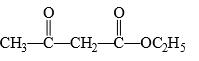Solution:
QUESTION: 4

Fehling’s solution can be used for distinguishing between:

Solution:
QUESTION: 5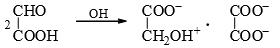​The above reaction can said to be an example of:

Solution:
QUESTION: 6

Ninhydrin has three keto groups, which of the keto group is expected to be hydrated most easily: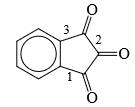Solution:
QUESTION: 7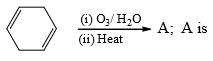Solution:
QUESTION: 8

Which of the following does not react with sodium bisulphate: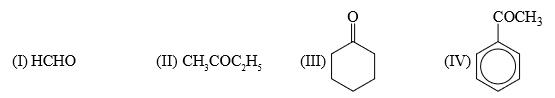Solution:
QUESTION: 9

Which of the following reagent can be used for carrying out the reaction outlined below: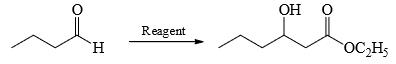Solution:
QUESTION: 10

Which of the following statement is false:

Solution:
QUESTION: 11

Which of the following compounds is not formed in iodoform reaction of acetone?

Solution:
QUESTION: 12

Which of the following is not formed in Iodoform reaction:

Solution:
QUESTION: 13

The major product formed in the following reaction is: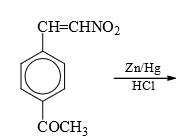Solution:
QUESTION: 14

The major product formed in the following reaction is: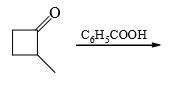Solution:
QUESTION: 15

Which of the following statement is true:

Solution:
QUESTION: 16

What is the main product whenSolution:
QUESTION: 17

Predict the nature of end product in the following reaction: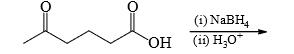Solution:
QUESTION: 18

Salicylic acid is treated with bromine under two different conditions: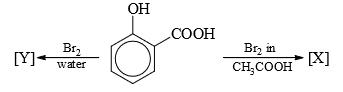Predict the nature of [X] and [Y] in the following reactions

Solution:
QUESTION: 19

A carboxylic acid can best be converted into acid chloride by using:

Solution:
QUESTION: 20

Which of the following does not reduce C6H5NO2 to aniline:

Solution:
QUESTION: 21

What could be the reagent for the following reaction:​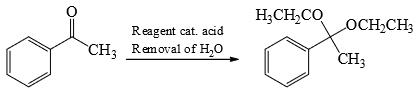Solution:
QUESTION: 22

What could be the product for the following reaction: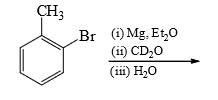Solution:
QUESTION: 23

Which ester will not give a good yield of the Claisen condensation product with NaOEt in EtOH?

Solution:

The Claisen condensation is reversible and it is formation of a stabilized enolate of the product which leads to a high yield at equilibrium. When the product cannot give a stabilized enolate, the yield will be poor.

QUESTION: 24

What could be the product for the following reaction: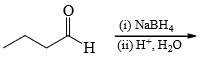Solution:
QUESTION: 25

What could be the product for the following reaction: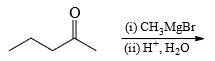Solution:
QUESTION: 26

What could be the product for the following reaction: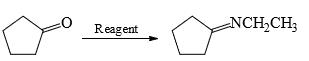Solution:
QUESTION: 27

The major product formed in the following reaction is: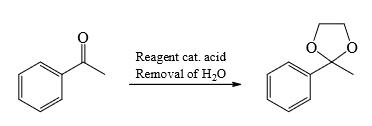Solution:
QUESTION: 28

The major product formed in the following reaction is: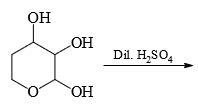Solution:
QUESTION: 29

The major product formed in the following reaction is: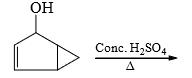Solution:
QUESTION: 30

The major product formed in the following reaction is: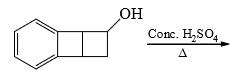Solution: